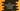# C++ example program to reverse a number# Introduction :

In this tutorial, we will learn how to reverse a number in C++ using one loop. We will use one while loop to reverse the number. The program will read the number as input from the user, reverse it and print it to the user.

## C++ program :

``````#include <iostream>
using namespace std;

int main()
{
//1
int num;
int rev = 0;
int rem = 0;

//2
cout << "Enter a number : "; cin >> num;

//3
while (num != 0)
{
rem = num % 10;
rev = rev * 10 + rem;
num /= 10;
}
//4
cout << "Reversed number : " << rev << endl;
}``````

### Explanation :

The commented numbers in the above program denote the step numbers below :

1. Create three integer variables: num to hold the user input number, rem to store remainder and rev to hold the final reversed number.
2. Ask the user to enter a number. Read the number and store it in the variable num.
3. Run one while loop. This loop is used to reverse the number. It finds the remainder of the number by dividing it by 10, i.e. on each step, it picks the rightmost digit of the number and adds this digit to the right of the reversed number variable rev.
4. Finally, print the variable to the user.

### Conclusion :

In this tutorial, we have learned how to reverse a number in C++. Try to go through the steps and drop one comment below if you have any queries.